# Python Program to Print an Inverted Star Pattern | Python code to create an inverted Pyramid start pattern

Beginners and experienced programmers can rely on these Best Java Programs Examples and code various basic and complex logics in the Java programming language with ease.

Python Program to Print the pattern of an Inverted Star: Given a number, the task is to print an Inverted Star Pattern in Python. In our python programming articles, you can also learn how to print a program of Inverted pyramid pattern and print inverted pyramid star pattern in python language.

Let’s see the examples of python program to print inverted star pattern from here

Example1:

Input:

given rows = 8

Output:

********
*******
******
*****
****
***
**
*

Example2:

Input:

given rows=10

Output:

**********
*********
********
*******
******
*****
****
***
**
*

Here is the program to print Inverted Star Pattern:

# python 3 code to print inverted star
# pattern

# n is the number of rows in which
# star is going to be printed.
n=11

# i is going to be enabled to
# range between n-i t 0 with a
# decrement of 1 with each iteration.
# and in print function, for each iteration,
# ” ” is multiplied with n-i and ‘*’ is
# multiplied with i to create correct
# space before of the stars.
for i in range (n, 0, -1):
print((n-i) * ' ' + i * '*')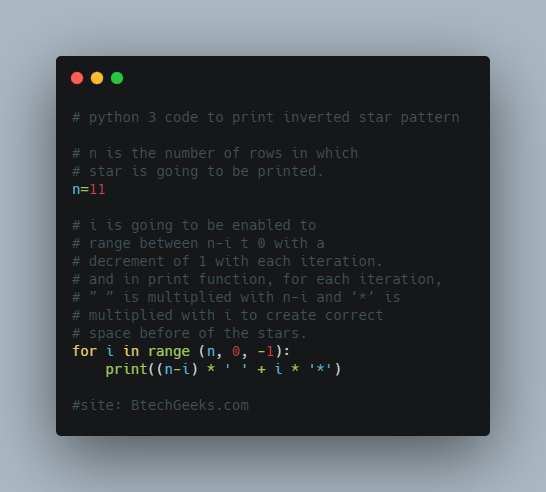## Program to Print an Inverted Star Pattern in Python

Below are the ways to print an Inverted Star Pattern in  Python :

Drive into Python Programming Examples and explore more instances related to python concepts so that you can become proficient in generating programs in Python Programming Language.

### 1)Using for loop printing the inverted star pattern in Python(Static Input)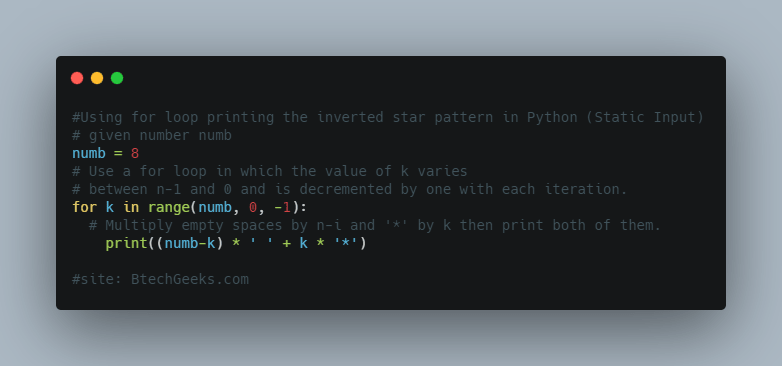Approach:

• Give the number as static and store it in a variable
• Use a for loop in which the value of k varies between n-1 and 0 and is decremented by one with each iteration.
• Multiply empty spaces by n-i and ‘*’ by k then print both of them.
• Exit of program.

Below is the implementation:

# given number numb
numb = 8
# Use a for loop in which the value of k varies
# between n-1 and 0 and is decremented by one with each iteration.
for k in range(numb, 0, -1):
# Multiply empty spaces by n-i and '*' by k then print both of them.
print((numb-k) * ' ' + k * '*')


Output:

********
*******
******
*****
****
***
**
*

Explanation:

• Give the number as static and stored it in  a variable numb
• The for loop allows I to range between n-1 and 0, with each iteration decrementing by one.
• To maintain proper star spacing, ” ” is multiplied by n-i and ‘*’ is multiplied by I for each iteration.
• The requisite pattern has been printed.

### 2)Using for loop printing the inverted star pattern in Python(User Input)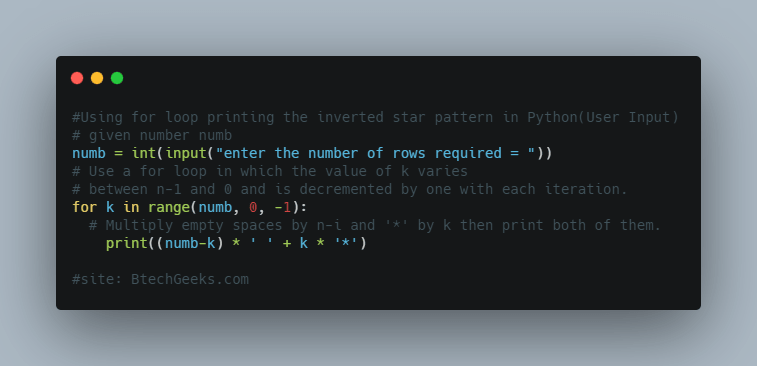Approach:

• Scan the given number using int(input()) and store it in a variable.
• Use a for loop in which the value of k varies between n-1 and 0 and is decremented by one with each iteration.
• Multiply empty spaces by n-i and ‘*’ by k then print both of them.
• Exit of program.

Below is the implementation:

# given number numb
numb = int(input("enter the number of rows required = "))
# Use a for loop in which the value of k varies
# between n-1 and 0 and is decremented by one with each iteration.
for k in range(numb, 0, -1):
# Multiply empty spaces by n-i and '*' by k then print both of them.
print((numb-k) * ' ' + k * '*')


Output:

enter the number of rows required = 10
**********
*********
********
*******
******
*****
****
***
**
*

### 3)Using while loop printing the inverted star pattern in Python(Static Input)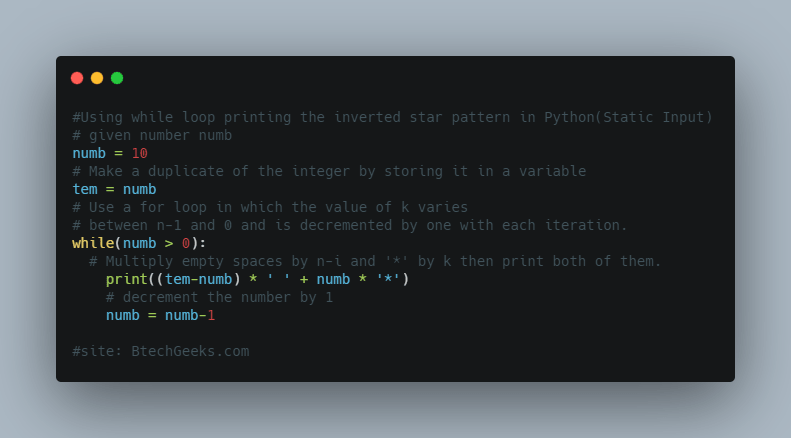Approach:

• Give the number as static and store it in a variable
• Using while loop iterate till number is greater than 0.
• Multiply empty spaces by tem-i and ‘*’ by numb then print both of them.
• Decrement the number by 1.
• The Exit of the program.

Below is the implementation:

# given number numb
numb = 10
# Make a duplicate of the integer by storing it in a variable
tem = numb
# Use a for loop in which the value of k varies
# between n-1 and 0 and is decremented by one with each iteration.
while(numb > 0):
# Multiply empty spaces by n-i and '*' by k then print both of them.
print((tem-numb) * ' ' + numb * '*')
# decrement the number by 1
numb = numb-1


Output:

**********
*********
********
*******
******
*****
****
***
**
*

### 4)Using while loop printing the inverted star pattern in Python(User Input)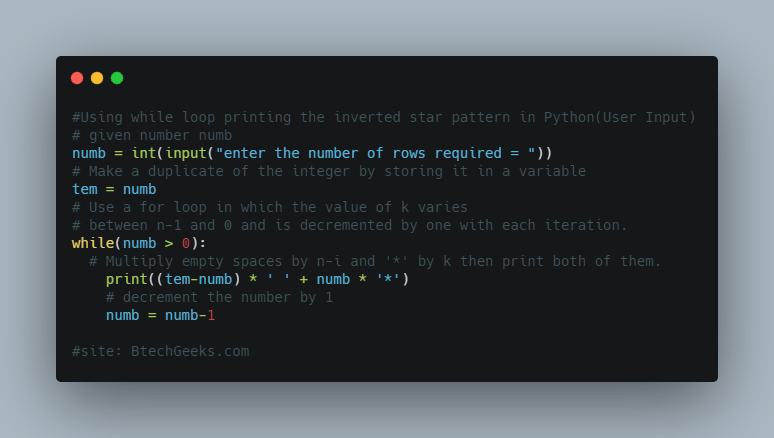Approach:

• Scan the given number using int(input()) and store it in a variable.
• Using while loop iterate till number is greater than 0.
• Multiply empty spaces by tem-i and ‘*’ by numb then print both of them.
• Decrement the number by 1.
• Exit of program.

Below is the implementation:

# given number numb
numb = int(input("enter the number of rows required = "))
# Make a duplicate of the integer by storing it in a variable
tem = numb
# Use a for loop in which the value of k varies
# between n-1 and 0 and is decremented by one with each iteration.
while(numb > 0):
# Multiply empty spaces by n-i and '*' by k then print both of them.
print((tem-numb) * ' ' + numb * '*')
# decrement the number by 1
numb = numb-1


Output:

enter the number of rows required = 10
**********
*********
********
*******
******
*****
****
***
**
*

### Programs to print inverted half pyramid using *

This instance is similar to an upright pyramid but that here we begin from the total number of rows and in each iteration, we decrease the number of rows by 1.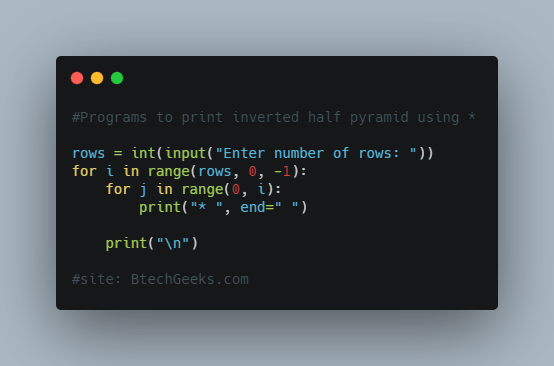Source Code:

rows = int(input("Enter number of rows: "))

for i in range(rows, 0, -1):
for j in range(0, i):
print("* ", end=" ")

print("\n")

output:

* * * * *
* * * *
* * *
* *
*

Related Programs: